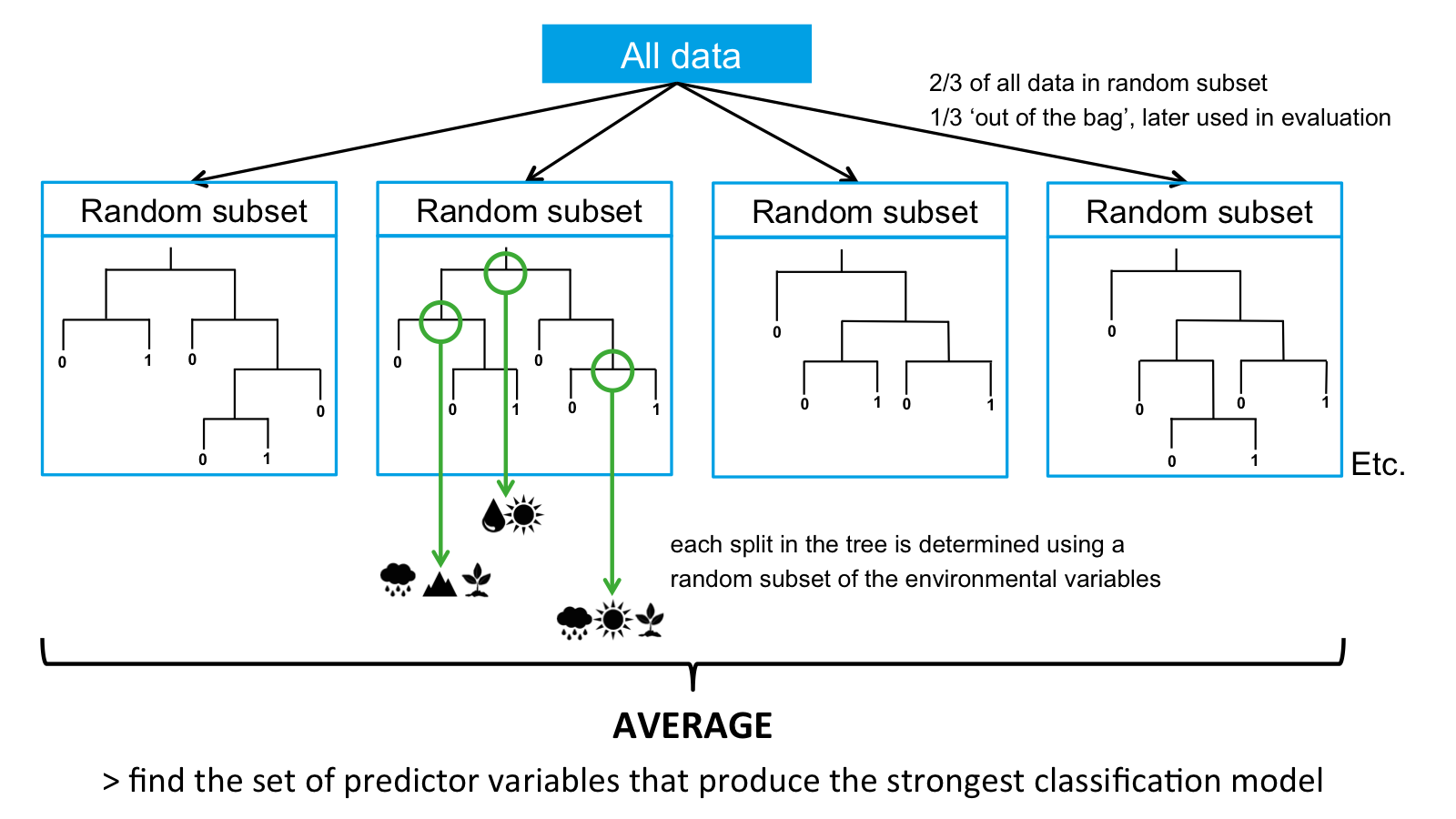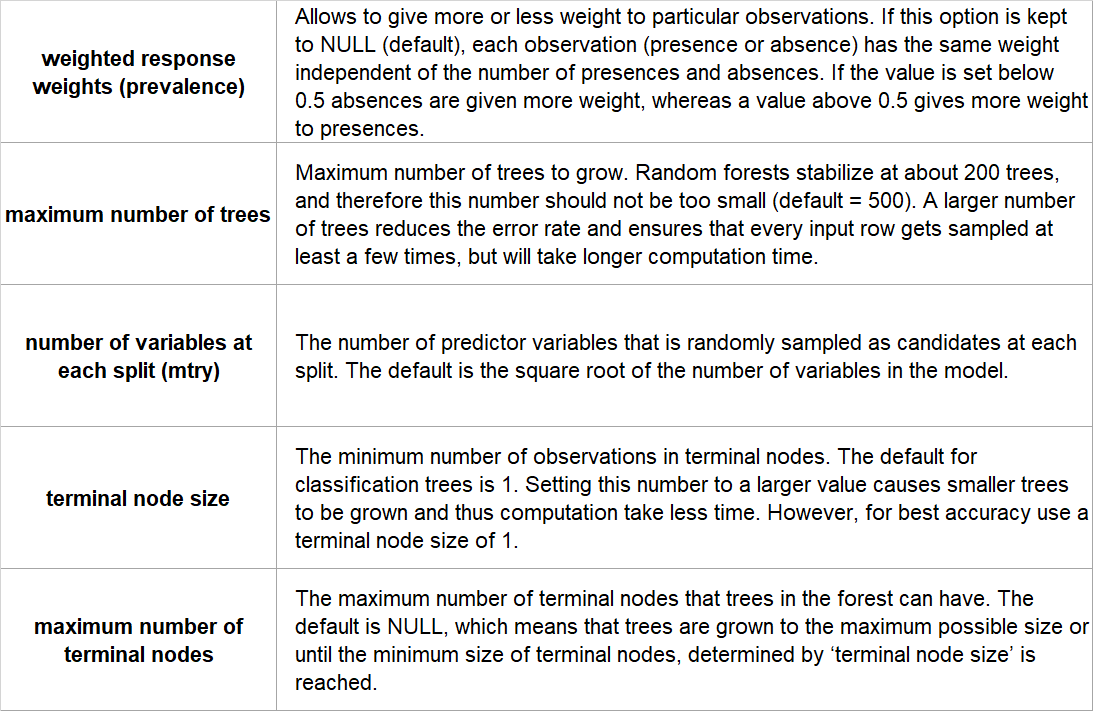### Introduction

Random Forests are an extension of single Classification Trees in which multiple decision trees are built with random subsets of the data. All random subsets have the same number of data points, and are selected from the complete dataset. Used data is placed back in the full dataset and can be selected in subsequent trees. In Random Forests, the random subsets are selected in a procedure called ‘bagging’, in which each data point has an equal probability of being selected for each new random subset. About two thirds of the total dataset is included in each random subset. The other third of the data is not used to build the trees, and this part is called the ‘out-of-the-bag’ data. This part is later used to evaluate the model.• One of the most accurate learning algorithms available
• It can handle many predictor variables
• Provides estimates of the importance of different predictor variables
• Maintains accuracy even when a large proportion of the data is missing

### Limitations

• Can overfit datasets that are particularly noisy
• For data including categorical predictor variables with different number of levels, random forests are biased in favor of those predictors with more levels. Therefore, the variable importance scores from random forest are not always reliable for this type of data

### Assumptions

No formal distributional assumptions, random forests are non-parametric and can thus handle skewed and multi-modal data as well as categorical data that are ordinal or non-ordinal.

Yes.

### Configuration options

BCCVL uses the ‘randomForest’ package, implemented in biomod2. The user can set the following configuration options:References

• Breiman L (2001) Random forests. Machine learning, 45(1): 5-32.
• Cutler DR, Edwards Jr TC, Beard KH, Cutler A, Hess KT, Gibson J, Lawler JJ (2007) Random forests for classification in ecology. Ecology, 88(11): 2783-2792.
• Franklin J (2010) Mapping species distributions: spatial inference and prediction. Cambridge University Press.
• Hastie T, Tibshirani R, Friedman J (2009) The elements of statistical learning: data mining, inference and prediction. Springer.